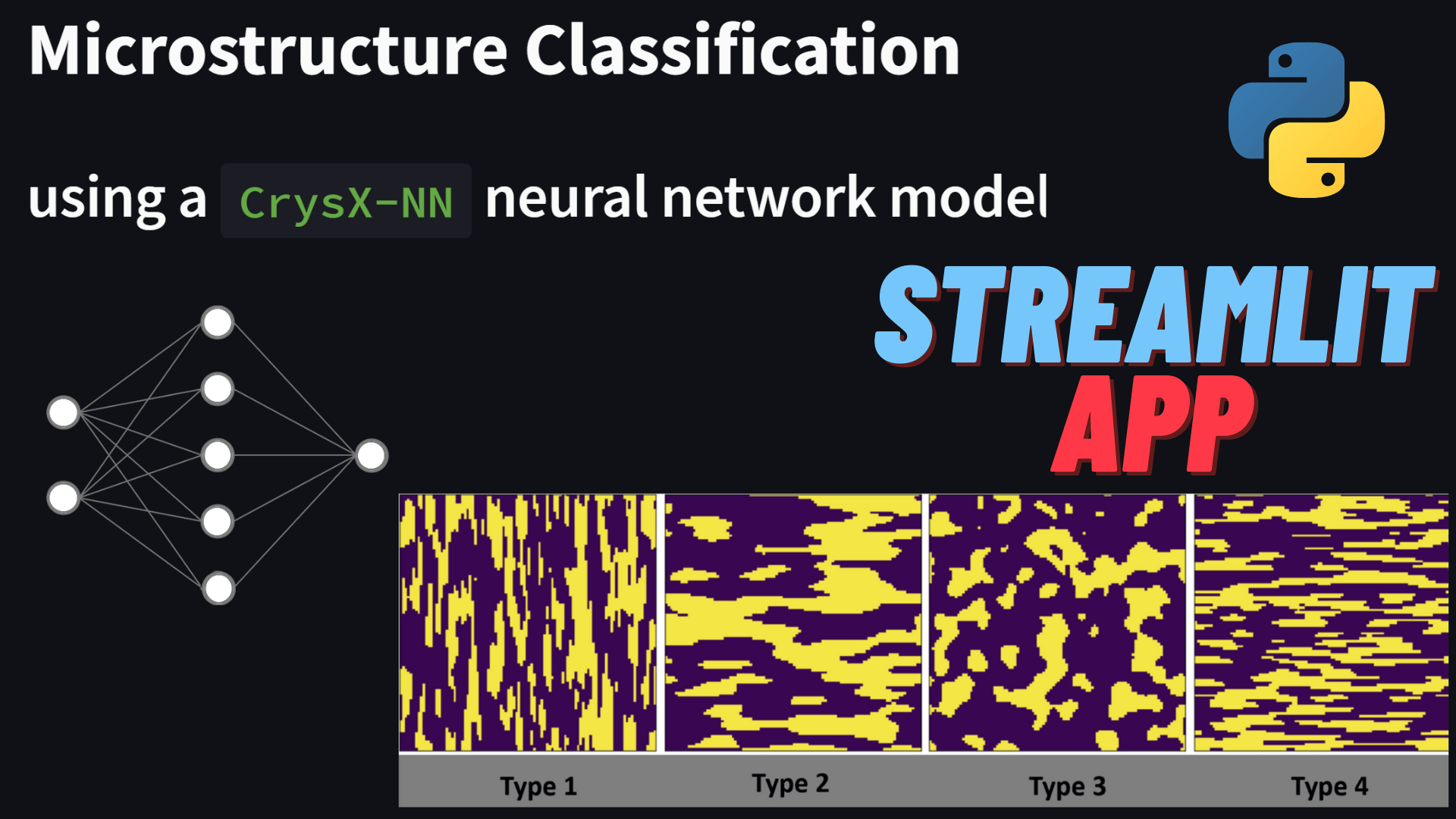# Microstructure Classification using Neural Networks – Streamlit AppIn this post, I demonstrate a Streamlit app that I have created that can be used to classify microstructures of different types.

I trained a neural network using CrysX-NN library, on synthetic microstructures of 4 types as shown in this notebook.

Check out the Streamlit App below (Source code is provided below the app)

https://share.streamlit.io/manassharma07/microstructure_classification_app/main/Microstructure_Classification_CrysX-NN_app.py

### Source Code:

```import streamlit as st
import matplotlib.pyplot as plt
import numpy as np
rng = np.random.default_rng()
from pymks import (
generate_multiphase,
)
from crysx_nn import network
import session_state
from streamlit.script_runner import RerunException
from streamlit.script_request_queue import RerunData

state = session_state.get(rndm_indx=5)

@st.cache
nInputs = 100*100 # No. of nodes in the input layer
neurons_per_layer = [500, 4] # Neurons per layer (excluding the input layer)
activation_func_names = ['ReLU', 'Softmax']
nLayers = len(neurons_per_layer)
nEpochs = 4
batchSize = 32 # No. of input samples to process at a time for optimization
# Create the crysx_nn neural network model
model = network.nn_model(nInputs=nInputs, neurons_per_layer=neurons_per_layer, activation_func_names=activation_func_names, batch_size=batchSize, device='CPU', init_method='Xavier')
# Load the preoptimized weights and biases
return model

@st.cache
def generate_microstructures(nSamples_per_type, width, height):
grain_sizes = [(30, 5), (10, 40), (15, 15), (5, 30)]
seeds = [10, 99, 4, 36]

data_synth = np.concatenate([
generate_multiphase(shape=(nSamples_per_type, width, height), grain_size=grain_size,
volume_fraction=(0.5, 0.5),
percent_variance=0.2,
# seed=seed
)
for grain_size, seed in zip(grain_sizes, seeds)
])
return data_synth

microstructure_data = generate_microstructures(20,100,100)
microstructures_labels = np.concatenate([np.ones(20)*0,np.ones(20)*1,np.ones(20)*2,np.ones(20)*3])

# @st.cache
def make_sidebar():
# st.sidebar.markdown("## [CrysX-NN](https://github.com/manassharma07/crysx_nn)")
st.sidebar.write('\n\n ## Neural Network Library Used')
st.sidebar.image('logo_crysx_nn-min.png')
st.sidebar.caption('https://github.com/manassharma07/crysx_nn')
st.sidebar.write('## Neural Network Architecture Used')
st.sidebar.write('1. **Inputs**: Flattened 100x100=10,000')
st.sidebar.write('2. **Hidden layer** of size **500** with **ReLU** activation Function')
st.sidebar.write('3. **Output layer** of size **4** with **Softmax** activation Function')
st.sidebar.write('Training was done for 4 epochs with Categorical Cross Entropy Loss function using [Stochastic Gradient Descent; learning rate=0.02; batch size of 32].')
st.sidebar.image('neural_network_visualization_25.png')
st.sidebar.caption('Nerual Network Schematic')

make_sidebar()

st.write('# Microstructure Classification')
st.write('## using a `CrysX-NN` neural network model')

st.write('### We have microstructures of 4 types:')

col1, col2, col3, col4 = st.columns(4)
col1.write('This  has 6 times more grain boundaries along the x-axis than the y-axis.')
col2.write('This has 4 times more grain boundaries along the y-axis than the x-axis.')
col3.write('This has the same number of grain boundaries along the x-axis as well as the y-axis.')
col4.write('This has 6 times more grain boundaries along the y-axis than the x-axis.')

st.image('microstructures_4_types-min.png')

st.write('### The following is a random `100x100` pixel picture of a microstructure of one of these 4 types.')

## The pyplot takes up a lot of screen
# fig, ax = plt.subplots()
# ax.imshow(microstructure_data[state.rndm_indx,:,:])
# ax.set_axis_off()
# st.pyplot(fig)

# So we use matplotlib to save an image instead
plt.imsave('processed_tensor.png',microstructure_data[state.rndm_indx,:,:], cmap='viridis')
st.image('processed_tensor.png', width=200)

st.write('### Can you guess which type is it?')

('Choose an option', 'Type 1', 'Type 2', 'Type 3', 'Type 4'), key=state.rndm_indx)

if option != 'Choose an option':
st.write('You selected:', option)
st.write('### True Type: '+str(int(microstructures_labels[state.rndm_indx])+1))
input = (microstructure_data[state.rndm_indx,:,:] - 0.5) / 0.5
input = input.reshape(1, 10000)
predictions = model.predict(input.astype(np.float32), loss_func_name='BCE')
# Get the maximum probability
certainty = np.max(predictions)
# Get the index of the maximum probability
output = np.argmax(predictions)
# st.write(predictions)
st.write('### Neural Network Prediction : '+str(int(output+1)))
st.write('### Certainty: '+ str(certainty*100)+' %')

if st.button('Next sample'):
state.rndm_indx = rng.integers(0, microstructure_data.shape)